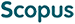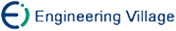黄海滨, 杨路明, 王建新, 陈建二, 李绍华. 基于子图的随机图点覆盖2度点核化研究[J]. 计算机研究与发展, 2009, 46(1): 31-40.
 引用本文: 黄海滨, 杨路明, 王建新, 陈建二, 李绍华. 基于子图的随机图点覆盖2度点核化研究[J]. 计算机研究与发展, 2009, 46(1): 31-40.Huang Haibin, Yang Luming, Wang Jianxin, Chen Jianer, Li Shaohua. Kernelization of 2-Vertex for Vertex Cover in Random Graphs Based on Subgraphs[J]. Journal of Computer Research and Development, 2009, 46(1): 31-40.
 Citation: Huang Haibin, Yang Luming, Wang Jianxin, Chen Jianer, Li Shaohua. Kernelization of 2-Vertex for Vertex Cover in Random Graphs Based on Subgraphs[J]. Journal of Computer Research and Development, 2009, 46(1): 31-40.## Kernelization of 2-Vertex for Vertex Cover in Random Graphs Based on Subgraphs

• 摘要: 点覆盖问题虽然可以在参数计算理论的架构内求精确解,但是目前在理论及应用上有一定的局限性.根据不同度的顶点之间及顶点与边的关系,提出随机图参数化点覆盖问题的d-核化可决策性及2度点三角形子图的计数方法;通过研究子图对顶点的共享关系,分析2度顶点核化过程中核及度分布演变的动态过程,得出随机图2度点核化强度与2度点概率关系及2度点核化可决策性的两个推论: 2度点核化算法对2度点分布概率约为0.75的随机图的核化强度最高;对顶点度概率分布为φ(x)的随机图的参数化点覆盖问题(G,k),当k小于某一与φ(x)有关的值时,它是2-核化可决策的.仿真结果证实,该理论能够把握2度点核化的内在机制,提供随机图上这一NP完全问题的求解方法,也为参数计算在已知度分布的一类不确定问题中的应用提供了可能.

Abstract: While the exact solution to vertex cover problem can be found within the frame of parameterized computation, there are some limits in the theory and practice, due to the lacking both in the analysis of algorithms mechanism and process and in the grasp of problems macroscopical and dynamic properties. On the basis of fixed-parameter tractability, the d-decision makable by way of kernelization (d-DMK) of the parameterized vertex cover problem of random graph is put forward and the counting method for triangle subgraphs with 2-degree vertex is also presented. According to the studies of the sharing relationship of the vertex between the subgraphs, the dynamic and evolvement mechanism of the kernel and the degree distribution in the process of 2-degree vertex kernelization are described, from which two deductions are drawn: the first states that the strength of the kernelization algorithm based on 2-degree gets its maximum in a random graph when the probability of its 2-degree vertex is about 0.75; the second states that the parameterized vertex cover problem (G, k) of random graph given by φ(x) is 2-DMK when k smaller than a given value relation to φ(x). The results of the emulation show that the theory can not only deal with the mechanism of the kernelization, but also offer an effective way to analyze such an NP-completeness problem in random graph and a new method to solve a class of uncertain problems with known degree distributions./下载:  全尺寸图片 幻灯片
• 分享
• 用微信扫码二维码

分享至好友和朋友圈